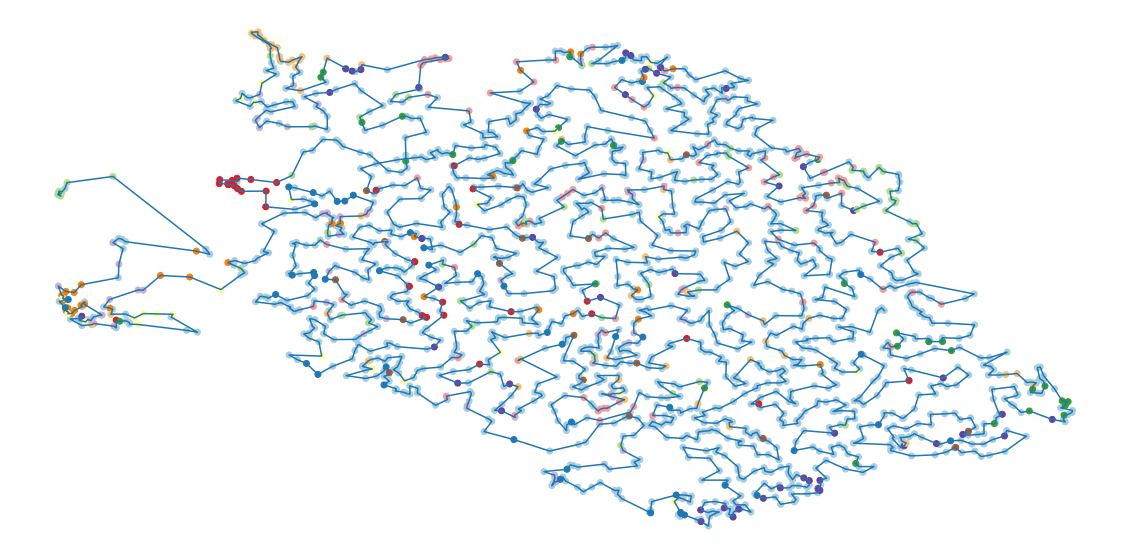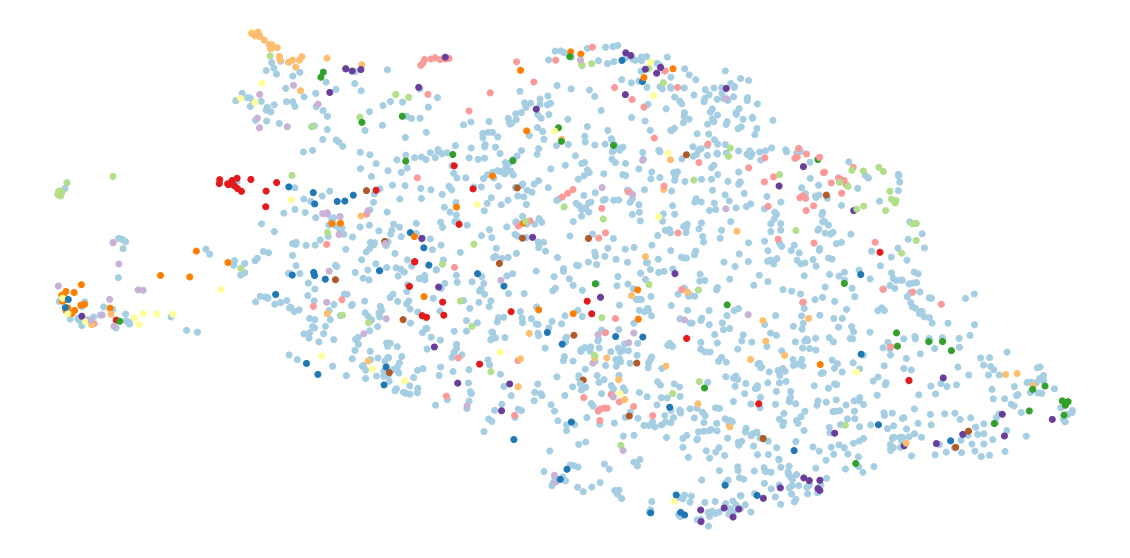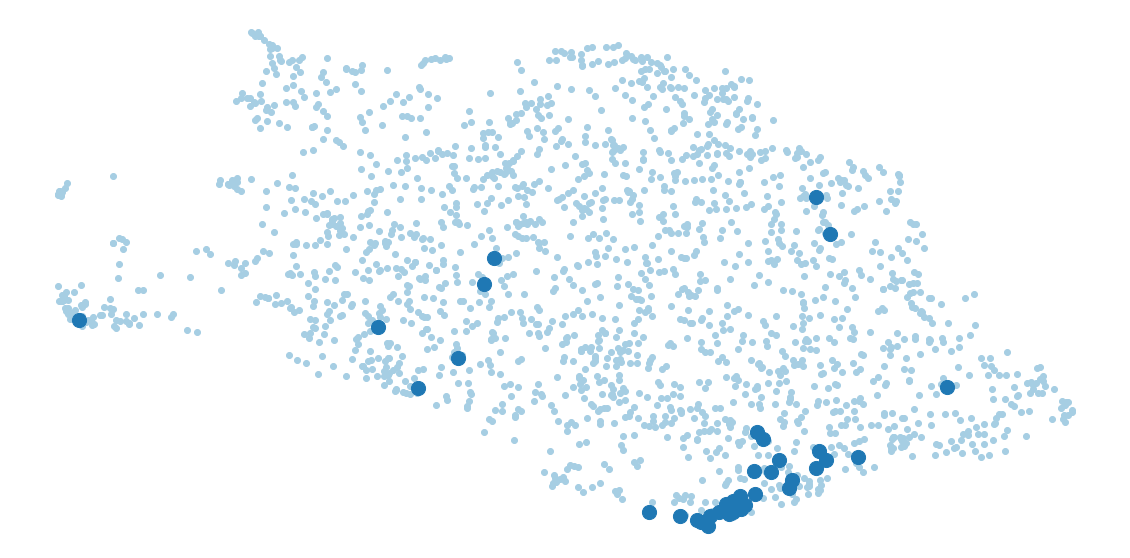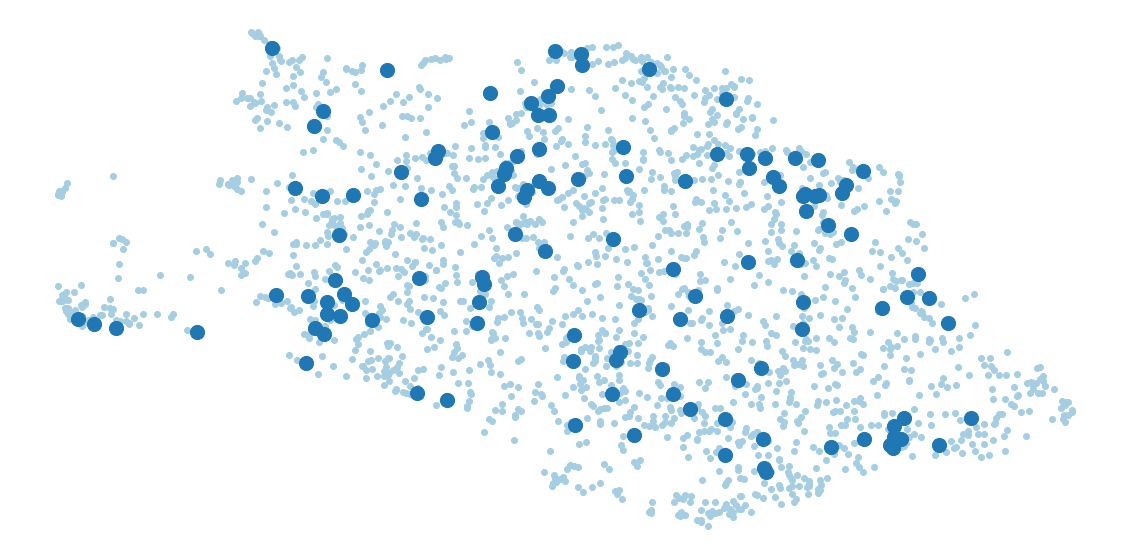# Vincent Zoonekynd's Blog

## Sun, 05 Jul 2020: How to sort a pile of research papers

When I have a large pile of papers to read, in electronic form, I do not want to read them in a random order: I prefer to read related papers together.

How can we sort hundreds of papers by topic?

Let us take the example of the CVPR conference (more than 1000 papers). We will proceed as follows:

- Retrieve the abstract of all the papers;

- Compute the embedding of each abstract, using the DistilBert pretrained model, from HuggingFace's Transformers library;

- Reduce the dimension to 2, to plot the data, using UMAP (we could use t-SNE instead);

- Find an ordering of the papers, i.e., a path, minimizing the total distance in the UMAP-reduced space (we could compute the distances in the original space, instead), using ortools's TSP solver.

The result can be a text file with the paper titles, links and abstract, in the order, or a pdf file with the first page of each paper, in the order (you can also read the begining of the introduction, and enjoy the plot on the first page, should there be one).Since this post is mostly code, here is its notebook version:

`https://colab.research.google.com/drive/1R_nAfhnlFsXPrLx4xtNrtXxp_2bV4yih`

## Tools

We will use

- WGet to retrieve the abstracts,

- BeautifulSoup to parse the HTML pages,

- HuggingFace's Transformers pretrained DistilBert model to process them,

- UMAP to reduce the dimension and plot the resulting cloud of points,

- ORTools to find a path in that cloud,

- qpdf to generate a single pdf file with the result.

`pip install requests bs4 transformers torch torchvision umap-learn matplotlib dill ortools `

## Raw data

First, retrieve the list of papers.

```wget -x -U Mozilla http://openaccess.thecvf.com/CVPR2020.py
wget -x -U Mozilla -r -l 1 -nc http://openaccess.thecvf.com/CVPR2020_workshops/menu.py```

Then, retrieve the abstracts of the papers

```for i in openaccess.thecvf.com/CVPR2020.py openaccess.thecvf.com/CVPR2020_workshops/*.py
do
get_urls "\$i"
done | sort | uniq | grep html |
perl -p -e 's#^[.][.]/##; s#^#http://openaccess.thecvf.com/#' > CVPR.urls
wget -U Mozilla -x -nc -i CVPR.urls```

(There is a non-standard command: "get_urls" extracts all the URLs in an HTML document. Writing it, as small Python script, is left as an exercise to the reader.)

## Parse the data

We now need to extract the titles and abstracts from the HTML files we have downloaded.

```import os, sys, re, time, math
from bs4 import BeautifulSoup

def LOG(*o):
print( time.strftime("%Y-%m-%d %H:%M:%S "), *o, file=sys.stderr, sep="", flush = True )

LOG( "1. Data" )

LOG( "URLs" )
with open("CVPR.urls") as f:
urls = [ u.replace("\n", "") for u in urls ]
LOG( f"  Found {len(urls)} URLs" )

LOG( "Parse the HTML to get the abstracts" )
d = []
missing = []
for i,u in enumerate(urls):
LOG( f"[{i+1}/{len(urls)}] {u}" )
filename = re.sub("^https?://", "", u)
if not os.path.exists(filename):
LOG( f"WARNING: Missing file {filename}" )
missing.append(u)
continue
with open(filename) as f:
soup = BeautifulSoup(html)

title = soup.find( "div", {"id": "papertitle"} )
title = title.text.strip()

authors = soup.find( "div", {"id": "authors"} )
authors = authors.find("b").text
authors = authors.split(",")
authors = [ x.strip() for x in authors ]

abstract = soup.find( "div", {"id": "abstract"} )
abstract = abstract.text
abstract = abstract.strip()

pdf = soup.find_all("a")
pdf = [ a for a in pdf if 'href' in a.attrs ]
pdf = [ a['href'] for a in pdf ]
pdf = [ a for a in pdf if a.endswith(".pdf") ]
base = re.sub("^(.*)/(.*?)\$", r"\1/", u)
pdf = [ ( ( base + a ) if a.startswith("..") else a ) for a in pdf ]
for _ in range(3):
pdf = [ re.sub("/[^/]+/[.][.]/", "/", a) for a in pdf ]

workshop = "paper"
if re.match("^.*/html/w[0-9]+/.*\$", u):
workshop = re.sub("^.*/html/(w[0-9]+)/.*\$", r"\1", u)

d.append( {
"title":    title,
"authors":  authors,
"workshop": workshop,
"pdf":      pdf,
"abstract": abstract,
} )

with open("cvpr_2020_papers.urls", "w") as f:
for u in d:
f.write( u['pdf'] + "\n" )

if len(missing) > 0:
LOG( f"A few files ({len(missing)}) were missing: " )
for u in missing:
LOG( f"  {u}" )```

## PDF files

If you want to download all the papers (it took 3 hours and almost 5GB on disk):

`wget -U Mozilla -x -nc -i cvpr_2020_papers.urls  `

## Compute the embedding of each abstract

BERT gives a contextual embedding of each word in a text. There is an additional token added at the begining, which gives an embedding of the whole text: that is what we want.

```LOG( "2. Sentence embeddings" )

LOG( "HuggingFace Transformers" )
import torch
import numpy as np
from transformers import DistilBertTokenizer, DistilBertModel

tokenizer = DistilBertTokenizer.from_pretrained('distilbert-base-cased')
model     =     DistilBertModel.from_pretrained('distilbert-base-cased')

def sentence_embedding(text):
## Code from: https://stackoverflow.com/questions/59030907/nlp-transformers-best-way-to-get-a-fixed-sentence-embedding-vector-shape
token_ids = tokenizer.encode(text)
token_ids = token_ids[:tokenizer.max_len]
#LOG( f"{[tokenizer._convert_id_to_token(idx) for idx in token_ids]}" )
token_ids = torch.tensor(token_ids).unsqueeze(0)   # unsqueeze token_ids because batch_size=1
output = model(token_ids).squeeze()
cls_out = output.detach().numpy()
return cls_out

LOG( "Compute embeddings [LONG]" )
## We process the abstracts one by one: it would be faster with minibatches
for i,r in enumerate(d):
LOG(f"[{i+1}/{len(d)}] {r['title']}" )
r['embedding'] = sentence_embedding( r['abstract'] )

LOG( "Save the data" )
import dill as pickle
with open( "cvpr.pickle", 'wb') as file:
pickle.dump( d, file )```

## Visualization

Let us use UMAP to reduce the dimension and plot the embedding. The colours correspond to the workshops, i.e., papers on a similar topic. Some of them cluster (the clearly visible clusters correspond to "medicine", "sports", "animal behaviour", "agriculture", "road traffic", etc.) but many do not.

```LOG( "Read the data" )
import dill as pickle
with open( 'cvpr.pickle', 'rb') as file:

LOG( "3. Visualization" )

import pandas as pd
import matplotlib
import matplotlib.pyplot as plt
data = np.array( [ x['embedding'] for x in d ] )

colours = pd.Categorical( [u['workshop'] for u in d] ).codes
palette = matplotlib.cm.Paired.colors
colours = [ palette[ i % len(palette) ] for i in colours ]

LOG( "UMAP" )
from umap import UMAP
xy = UMAP().fit_transform(data)
assert xy.shape == len(d)
fig, ax = plt.subplots()
ax.scatter(xy[:,0], xy[:,1], color=colours)
plt.show()```To check if nearby points are on the same topic, we can add the titles of the papers, and check them manually.

```import plotly.express as px
p = pd.DataFrame( {
'x': xy[:,0],
'y': xy[:,1],
'workshop': [u['workshop'] for u in d],
'title':    [u['title']    for u in d],
} )
fig = px.scatter(
p,
x="x", y="y", color="workshop",
hover_data={'title':True, 'x': False, 'y': False, 'workshop': False}
)
fig.layout.update(showlegend=False)
fig.show()```

We can also check if papers with a given keyword appear close together. They sometimes do.

```word = 'NAS' # Netwotk architecture search
#word = 'medical'

palette = matplotlib.cm.Paired.colors
i = [ word in u['title'] + u['abstract'] for u in d ]
fig, ax = plt.subplots( figsize=(20,10) )
ax.scatter(xy[:,0], xy[:,1], color=palette)
ax.scatter(xy[i,0], xy[i,1], color=palette, s = 200)
plt.show()```But not always...

```#word = 'unsupervised'
word = 'GAN'
#word = 'generative'
#word = 'Wasserstein'
#word = 'hyperbolic'

palette = matplotlib.cm.Paired.colors
i = [ word in u['title'] + u['abstract'] for u in d ]
fig, ax = plt.subplots( figsize=(20,10) )
ax.scatter(xy[:,0], xy[:,1], color=palette)
ax.scatter(xy[i,0], xy[i,1], color=palette, s = 200)
plt.show()```## Traveling Salesman Problem (TSP)

To order the papers, let us find a path in that cloud of points, visiting each point once, with the shortest total length. This is an instance of the traveling salesman problem (TSP): we can use ortools to solve it.

```LOG( "4. TSP: find a path, going through all the points, with the lowest possible total distance, in the 2-dimensional UMAP space" )
from ortools.constraint_solver import pywrapcp
from ortools.constraint_solver import routing_enums_pb2
from scipy.spatial import distance_matrix
np.core.arrayprint.set_printoptions(linewidth = 160)```

To compute the distances, we could use the Euclidean distances in the 2-dimensional space from the UMAP algorithm, the Euclidean distances in the original embedding, or the cosine similarity in the original embedding space. Cosine similarity may be preferable, but the 2-dimensional space will give prettier plots.

```LOG( "Distance matrix" )
di = distance_matrix(xy, xy)
#di = distance_matrix(data, data)

LOG( "The solver expects integers: make sure rounding does not discard any information" )
di = np.round(1000*di)```

The textbook traveling salesman problem asks for a closed path -- we want an open one. We can reduce the open TSP to the closed one by adding another point, at the same distance to all the others.

```LOG( "The Traveling Salesman Problem (TSP) looks for a closed path, but I want an open one." )
LOG( "Add an extra node, 0, at the same distance to all the other nodes -- i.e., add a row and a column of zeros" )
n = di.shape
di = np.hstack( [ np.zeros(n).reshape((n,1)), di ] )
di = np.vstack([ np.zeros(n+1), di ])```

We can now solve the optimization problem (this code closely follows the ortools documentation).

```LOG( "Describe the optimization problem (ortools API)" )
manager = pywrapcp.RoutingIndexManager(n+1, 1, 0)  # n+1 nodes, 1 vehicle, start and end at node 0
routing = pywrapcp.RoutingModel(manager)
def distance_callback(i, j):
return di[ manager.IndexToNode(i), manager.IndexToNode(j) ]
transit_callback_index = routing.RegisterTransitCallback(distance_callback)
routing.SetArcCostEvaluatorOfAllVehicles(transit_callback_index)

## Greedy
search_parameters = pywrapcp.DefaultRoutingSearchParameters()
search_parameters.first_solution_strategy = (routing_enums_pb2.FirstSolutionStrategy.PATH_CHEAPEST_ARC)

## Local search
search_parameters = pywrapcp.DefaultRoutingSearchParameters()
search_parameters.first_solution_strategy = (routing_enums_pb2.FirstSolutionStrategy.PATH_CHEAPEST_ARC)
#search_parameters.first_solution_strategy = (routing_enums_pb2.FirstSolutionStrategy.CHRISTOFIDES)
search_parameters.local_search_metaheuristic = (routing_enums_pb2.LocalSearchMetaheuristic.GUIDED_LOCAL_SEARCH)
#search_parameters.local_search_metaheuristic = (routing_enums_pb2.LocalSearchMetaheuristic.SIMULATED_ANNEALING)
search_parameters.time_limit.seconds = 5 * 60
search_parameters.log_search = True

LOG( "Solve the problem [LONG]" )
solution = routing.SolveWithParameters(search_parameters)

LOG( "Extract the solution" )
def get_routes(solution, routing, manager):
"""Get vehicle routes from a solution and store them in an array."""
# Get vehicle routes and store them in a two dimensional array whose
# i,j entry is the jth location visited by vehicle i along its route.
routes = []
for route_nbr in range(routing.vehicles()):
index = routing.Start(route_nbr)
route = [manager.IndexToNode(index)]
while not routing.IsEnd(index):
index = solution.Value(routing.NextVar(index))
route.append(manager.IndexToNode(index))
routes.append(route)
return routes

route = get_routes(solution, routing, manager)
route = np.array(route[1:-1])-1```

Here is the path.

```LOG( "Plot the solution" )
fig, ax = plt.subplots()
ax.plot( xy[route,0], xy[route,1] )
ax.scatter(xy[:,0], xy[:,1], color=colours)
ax.set_axis_off()
plt.show()```## Results

To use this route through the papers, we can put all the abstracts in a text file, in the order, to read them.

```LOG( "5. Results" )

LOG( "Print all the abstracts, in this order" )

with open("cvpr_2020_abstracts.txt", "w") as f:
for i,j in enumerate(route):
f.write( d[j]['title'] + "\n" )
f.write( d[j]['pdf'] + "\n" )
f.write( d[j]['abstract'] + "\n\n" )

file = re.sub("[^A-Za-z0-9]","-", d[j]['title'])
file = f"{i+1:04}-{file}.pdf"
print( f"{i+1} [{d[j]['type']}] {d[j]['title']}" )
print( d[j]['abstract'] )
if d[j]['slides'] is not None:
print( d[j]['slides'] )
if d[j]['video'] is not None:
print( d[j]['video'] )
print( "\n" )```

Another way of using this order is to print the papers in this order. I usually only print the first page of each paper, to read the abstract, part of the introduction, and look at the figure on the first page when there is one.

```LOG( "When the number of papers is more reasonable (a few hundreds), I download them all and look at the first page of each" )

missing = []
first_pages = []
with open( "cvpr_2020_pdf.sh", "w" ) as f:
for i,j in enumerate(route):
url  = d[j]['pdf']
file = re.sub( r"^https?://", "", url )
if not os.path.exists(file):
LOG( f"Missing: {file}" )
missing.append( url )
continue
new_file = f"pdf/{i:05}.pdf"
first_pages.append( new_file )
#f.write( f"qpdf {file} --pages . 1 -- {new_file}\n" )
f.write( f"qpdf --empty --pages {file} 1 -- {new_file}\n" )
f.write( "qpdf --empty --pages/pdf/*.pdf -- out.pdf\n" )

LOG( "Done." )```

What I often do is slightly trickier than that, especially if the papers are not typeset with the same template: I also remove the margins of each of the pdf files.

```pdfcrop1() {
input="\$1"
bbox="\$2"
output="\$1"_cropped.pdf
if test "\$bbox" = ""
then
bbox=\$(
gs -dSAFER -dNOPAUSE -dBATCH -sDEVICE=bbox "\$input" 2>&1 |
perl -MList::Util=max,min -n -e '
BEGIN { \$x1 = 1e9; \$y1 = 1e9; \$x2 = 0; \$y2 = 0; \$first = 1;}
next unless m/HiResBoundingBox:\s+([0-9.]+)\s+([0-9.]+)\s+([0-9.]+)\s+([0-9.]+)/;
if( \$first ) { \$first = 0; chomp; print STDERR \$_ . " [skipped]\n"; next; }
print STDERR \$_;
\$x1 = min \$x1, \$1;
\$y1 = min \$y1, \$2;
\$x2 = max \$x2, \$3;
\$y2 = max \$y2, \$4;
END {
\$margin = 10;
\$x1 -= \$margin; \$x2 += \$margin;
\$y1 -= \$margin; \$y2 += \$margin;
print "\$x1 \$y1 \$x2 \$y2";
}
'
)
fi
echo "Setting bounding box to: \$bbox"
gs \
-o "\$output" \
-sDEVICE=pdfwrite \
-c "[/CropBox [\$bbox] /PAGES pdfmark" \
-sPAPERSIZE=a4 \
-dFIXEDMEDIA \
-dPDFFitPage \
"\$input"
echo "Input:  \$input"
echo "Output: \$output"
echo "Bounding box: \$bbox"
pdfinfo "\$output"
}```

## Conclusion

While the order found is not perfect (papers next to one another in the order have similar topics, but papers on similar topics need not appear close to one another), it is better than a random or alphabetic order.

Possible improvements include:

- An objective measure of how good the result is

- Other language models (I used DistilBert);

- A language model fine-tuned on arxiv abstracts (arxiv, contrary to SSRN, has an API: it is straightforward to retrieve a very large number of abstracts);

- Alternatives to t-SNE and UMAP (in particular, we could fine-tune the hyperparameters to have more clearly visible clusters)

```  LargeVis    (not pip-installable?)
Trimap      pip install trimap   https://pypi.org/project/trimap/
VAE         pip install cve      https://maxfrenzel.com/articles/compression-vae
Mapper      pip install mapper
```

- Other TSP solvers

- Other ways of ordering elements (there is a long list in the "seriation" R package)

```Getting Things in Order: An Introduction to the R Package seriation
M. Hahsler et al. (Journal of statistical software, 2008)
https://cran.r-project.org/web/packages/seriation/vignettes/seriation.pdf```

posted at: 18:47 | path: /ML | permanent link to this entry